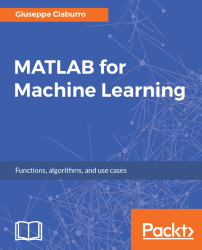•#### MATLAB for Machine Learning#### Overview of this book

MATLAB is the language of choice for many researchers and mathematics experts for machine learning. This book will help you build a foundation in machine learning using MATLAB for beginners. You’ll start by getting your system ready with t he MATLAB environment for machine learning and you’ll see how to easily interact with the Matlab workspace. We’ll then move on to data cleansing, mining and analyzing various data types in machine learning and you’ll see how to display data values on a plot. Next, you’ll get to know about the different types of regression techniques and how to apply them to your data using the MATLAB functions. You’ll understand the basic concepts of neural networks and perform data fitting, pattern recognition, and clustering analysis. Finally, you’ll explore feature selection and extraction techniques for dimensionality reduction for performance improvement. At the end of the book, you will learn to put it all together into real-world cases covering major machine learning algorithms and be comfortable in performing machine learning with MATLAB.
Title PageCreditsForewordwww.PacktPub.comCustomer FeedbackPrefaceFree Chapter
Getting Started with MATLAB Machine LearningImporting and Organizing Data in MATLABFrom Data to Knowledge DiscoveryFinding Relationships between Variables - Regression TechniquesPattern Recognition through Classification AlgorithmsIdentifying Groups of Data Using Clustering MethodsSimulation of Human Thinking - Artificial Neural NetworksImproving the Performance of the Machine Learning Model - Dimensionality ReductionMachine Learning in Practice## Summary

In this chapter, we learned how to perform an accurate cluster analysis in the MATLAB environment. First, we explored how to measure similarity. We learned concepts such as proximity between elements, similarity and dissimilarity measures, and Euclidean, Minkowski, Manhattan, and cosine distance metrics. We looked at a couple of methods for grouping objects: hierarchical clustering and partitioning clustering. In the first method, clusters are constructed by recursively partitioning the instances in either a top-down or bottom-up fashion. The second one decomposes a dataset into a set of disjoint clusters.

We discovered hierarchical clustering in MATLAB using the `pdist`, `linkage`, and `cluster` functions. These functions perform agglomerative clustering. We learned how to calculate the distance between the objects through the `pdist` function. To determine the proximity of objects to each other, we used the linkage function. With the cluster function, we cut the ramifications from the bottom...